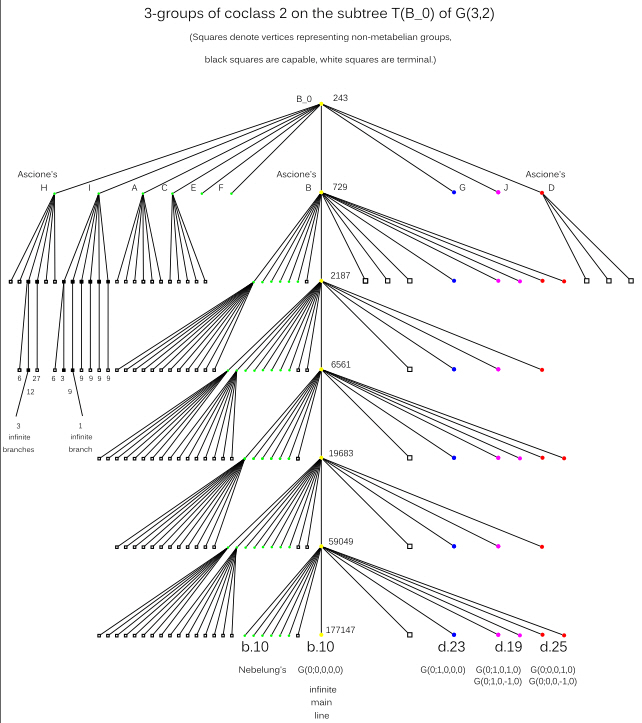# Oujda Research Project 2011

## The Subtree T(B0) of the Coclass Graph G(3,2)

The vertices of the coclass graph G(3,2) represent all finite 3-groups G of coclass cc(G)=2.
G(3,2) consists of finitely many sporadic groups and 16 coclass trees,
each with a single infinite main line and a structural periodicity.

The descendants of B0 form one of these trees, T(B0).
They are characterized by the isomorphism invariant ε = 2, indicating that
two of the abelianizations Mi/M'i (1 ≤ i ≤ 4)
of their maximal subgroups are of type (3,3,3).

Succinct presentation of structural details:
The pathology of this tree is due to the following fact:
whereas in other trees capable vertices represent metabelian groups (circles),
we now have also capable non-metabelian groups (black squares).
As usual, several terminal vertices represent non-metabelian groups (white squares).

With the aid of colors we characterize the transfer types:
the main line of type b.10 (0043) (yellow, with center of type (3,3)) and coclass families of types b.10 (0043) (green, with cyclic center of order 3),
d.23 (1043) (blue), d.19 (4043) (magenta), and d.25 (2043) (red).Applications in class field theory:

None of the vertices of the tree T(B0)
can be realized as the second 3-class group of a quadratic number field K = Q(sqrt(D)),
that is, the Galois group Gal(F32(K)|K) of the second Hilbert 3-class field.
Since the occurring transfer types b.10 (0043), d.23 (1043), d.19 (4043), and d.25 (2043)
contain at least one zero, which indicates a total transfer, the complex quadratic base fields are excluded.
On the other hand, a zero on the second position implies an odd coclass r ≥ 3 in the case of a real quadratic base field.

 * Web master's e-mail address: contact@algebra.at *